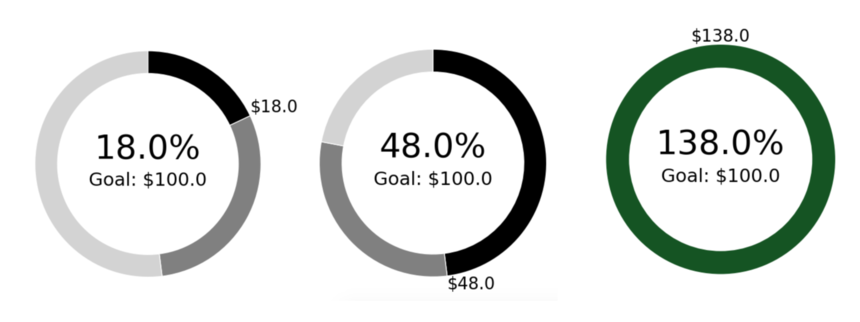# Radial Bar Chart with Pending PipelineRadial bar charts are a great way to visualize progress charts - but sometimes it's helpful to be able to visualize that progress with the additional context of more pipeline as well.

Input: you will need to provide the chart maker function with at least two values (progress and goal - you will just need to edit the very bottom section of this code block). You can also specify a pipeline value if you have one.

```current = float(101) #float(df.iloc[0,0]
goal = float(100) #float(df.iloc[0,1]

# this is an optional parameter if you do have pipeline
pipeline = float(30) #float(df.iloc[0,1]```

Output: a single ring that denotes progress towards goal (optionally with pipeline as well). Anything past 100% will result in a color change indicating the goal has been reached.

Full Code Block:

```# Periscope automatically imports the output from the SQL editor above into the dataframe when you add Python analysis.
# Filters applied in the SQL workflow above will update Python based analysis when applied.

#######################################################################
###                                                        LIBRARIES                                                            ###
#######################################################################
import pandas as pd
import matplotlib.pyplot as plt
import numpy as np

#######################################################################
###                                                    HELPER FUNCTIONS                                                ###
#######################################################################

#USE: Create an array structure for rings.
#INPUT: a df of row length 1 with the first column as the current metric value and the second colum is the target metric value
#OUTPUT: an aray of arrays representing each ring
def calculate_rings(first, second, pending):
if first < second and first + pending <= second:
rings=[[first, pending, second-first - pending]]
elif first < second and first + pending > second:
rings=[[first, second - first , 0]]
else:
rings = [[1, 0, 0]]
return rings

#USE: Determine if the label for the rotating number label should be left/center/right
#INPUT: a df of row length 1 with the first column as the current metric value and the second colum is the target metric value
#OUTPUT: the proper text alignment
def horizontal_aligner(first, second):
metric = 1.0 * first % second / second
if metric in (0, 0.5):
align = 'center'
elif metric < 0.5:
align = 'left'
else:
align = 'right'
return align

def vertical_aligner(first, second):
metric = 1.0 * first % second / second
if metric < 0.25:
align = 'bottom'
elif metric < 0.75:
align = 'top'
elif metric > 0.75:
align = 'bottom'
else:
align = 'center'
return align

#USE: Create a center label in the middle of the radial chart.
#INPUT: a df of row length 1 with the first column as the current metric value and the second column is the target metric value
#OUTPUT: the proper text label
percent = str(round(1.0*first/second*100,1)) + '%'
return plt.text(0,
0.2,
percent,
horizontalalignment='center',
verticalalignment='center',
fontsize = 40,
family = 'sans-serif')

#USE: Formats a number with the apropiate currency tags.
#INPUT: a currency number
#OUTPUT: the properly formmated currency string
def get_currency_label(num):
currency = ''
if num < 10**3:
currency = '\$' + str(num)
elif num < 10**6:
currency = '\$' + str(round(1.0*num/10**3,1)) + 'K'
elif num < 10**9:
currency = '\$' + str(round(num/10**6,1)) + 'M'
else:
currency = '\$' + str(round(num/10**9,1)) + 'B'

return currency

#USE: Create a dynamic outer label that servers a pointer on the ring.
#INPUT: a df of row length 1 with the first column as the current metric value and the second column is the target metric value
#OUTPUT: the proper text label at the apropiate position
currency = get_currency_label(first)

return plt.text(1.5 * np.cos(0.5 *np.pi - 2 * np.pi * (first /second)) if first < second else 0,
1.5 * np.sin(0.5 *np.pi - 2 * np.pi * first / second) if first < second else 1.5,
currency,
horizontalalignment=horizontal_aligner(first, second) if first < second else 'center',
verticalalignment=vertical_aligner(first, second) if first < second else 'bottom',
fontsize = 20,
family = 'sans-serif')

amount = 'Goal: ' + get_currency_label(second)
return plt.text(0,
-.1,
amount,
horizontalalignment='center',
verticalalignment='top',
fontsize = 22,family = 'sans-serif')

#######################################################################
###                                                    MAIN FUNCTION                                                        ###
#######################################################################

def chart(current, goal, pipeline=0, colors = ["black", 'gray', 'lightgray'], overachiever_color = '#0c561d', width = 5, height = 5, dpi = 100):

first = current
second = goal

# base styling logic
ring_width = 0.3

# set up plot
ring_arrays = calculate_rings(first, second, pipeline)
fig, ax = plt.subplots(figsize = (width,height), dpi = dpi)

if first  >= second:
outer_edge_color = None
inner_edge_color = 'white'
colors = [overachiever_color, 'white', 'white']
else:
outer_edge_color, inner_edge_color = ['white', None]

# plot logic

outer_ring, _ = ax.pie(ring_arrays,radius=1.5,
colors=colors,
startangle = 90,
counterclock = False)

plt.setp( outer_ring, width=ring_width, edgecolor=outer_edge_color)
ax.axis('equal')
plt.margins(0,0)
plt.autoscale('enable')

return plt

# TODO: EDIT these to reflect where the variables exist in your data frame

current = float(101) #float(df.iloc[0,0]
goal = float(100)  #float(df.iloc[0,1]

# this is an optional parameter if you do have pipeline
pipeline = float(30)  #float(df.iloc[0,1]

# call the function and output in periscope
periscope.output(chart(current, goal, pipeline,
colors = ["black", 'gray', 'lightgray'], overachiever_color = '#0c561d'))
#additional options (specify a color array of three colors) called color, matching current, pipeline, goal
#specify an overachiever color```

Shout out to Kyle Dempsey for surfacing this use case.